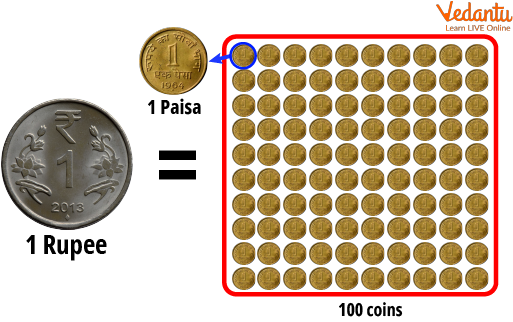Courses
Courses for Kids
Free study material
Offline Centres
More

# How to Convert Paise Into Rupees Using DecimalLast updated date: 23rd Nov 2023
Total views: 97.5k
Views today: 1.97k## Use of Decimal to Convert Paisa Into Rupees

In ancient times, people traded using gold and silver before there was anything known as money or currency. The word rupee, also called rupaya, rupaye or rupiya is derived from a Sanskrit word that means Silver Coin. The rupee is denoted as ‘INR,’ an abbreviated form of ‘Indian Rupee.’ Rupees are made up of 100 paise. These 100 paise in each rupee are used as 100 parts of a fraction or decimal. There are many ways to represent this distinction and convert it from one to another.

## Representation of Money

Indian Currency, the rupee, has many forms of representation. It can be denoted as ‘INR,’ the Indian rupee or with the symbol of rupees ₹. When the symbol of rupee ₹ cannot be used, one can use the abbreviated form of rupees, that is, ‘Rs.’ Rupees are divided into one hundred paise that can be represented in decimal forms.Representation of 100 paise makes 1 rupee

## Use of Decimal to Represent Money

Any currency can be represented using decimals since they are all multiples of 100.

Like, $1 = 100 cents Similarly, ₹1 = 100 paise ∴ 1 paisa = ₹$0.01$ ### How to Use Decimals to Represent Money? If one wants to describe the money they have in rupees and paise, they must not say they have ‘x’ rupees and ‘y’ paise. Decimals come in handy in such situations. ### Solved Examples of Using Decimals to Represent Money: Q1. How to write ₹15 and 25 Paise? Ans. ₹15.25 Q2. How to write ₹45 and 67 Paise? Ans. ₹45.67 Q3. How much is ₹34.89? Ans. ₹34 and 89 Paise ## How to Convert Paise Into Rupee Using Decimals? To convert paise into rupees or vice versa, one must be familiar with the conversion number line. Moving two places to the right makes the unit smaller and increases the face value by 100 folds simultaneously. Similarly, moving two places to the left makes the unit bigger but reduces the face value 100 times. For Example, ₹100.0 = 10000 paise. Here, the face value of the number increased from 100 to 10000, whereas the unit became smaller, from rupees to paise. Similarly, 1000 paise = ₹ 10.00. Here, the face value of the number decreased, whereas the unit's value increased. Therefore, to convert paise into rupees, one must remember to move two decimal places to the left, which can also be seen as multiplying the number by 100. ∴ Rupees x 100 = Paise &$\frac{Paise}{100}$= Rupees ### Solved Examples of Converting Paise Into Rupees Q1. How to write ₹25 and 250 Paise? Ans. 1 rupee = 100 paise ∴ 250 paise can be divided into 2 rupees and 50 paise ∴ We have$25+2$rupees and 50 paise ∴ We can say we have ₹27.50 Q2. How many rupees is 50000 paise? Ans.$\frac{Paise}{100}$= Rupees ∴$\frac{50000}{100}$= ₹500 Q3. Convert ₹ 34.78 into paise Ans.$34.78\times 100=3478$paise ## FAQs on How to Convert Paise Into Rupees Using Decimal 1. Is the rupee considered a word? Yes, the rupee is considered a valid word in the English Language. 2. Is the rupee used as a currency in any other country? Yes, the rupee is used as a currency in the following countries: • India • Pakistan • Bhutan • Seychelles • Sri Lanka • Mauritius, • Nepal But, the abbreviation of ‘INR’ is only valid for the Indian Rupee. 3. Is there a sub-division of paisa? No, paisa is indivisible. It is the$\frac{1}{100}th\$ part of the rupee.

4. Are there any other sub-divisions of the rupee?

Yes. ₹1 = 16 anna = 64 pice. These subdivisions were discontinued to make calculations easier through the multiples of 100.

5. Where are commas put in the representation of rupees?

Commas are added in bigger valued numbers of thousands and above. The first comma is added 3 digits left from the decimal point in the thousandth place. The next is added two places on the left of the first comma.